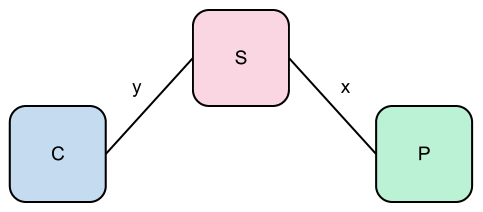# A Kind of Informal Introduction to π-Calculus

06 Jan 2013 pi-calculus

The π-Calculus is one of many approaches to concurrent computation by the means of formal modeling. Its purpose is to enable us to reason about concurrent processes in a disciplined fashion by manipulating expressions through formally defined algebraic rules.

Any exposition of the calculus will eventually introduce you to the primitive notions:

• Agents, which can be understood and even referred as processes.
• Actions, which are anything that can be done by an agent.
• Channels, which are links connecting agents, alowing them to communicate.
• Names, which are exchanged through channels.

The general idea is that we have a set of agents connected to each other through channels in some sort of network. These agents then use these channels communicate to each other by exchanging names.

The role of communication is central to the π-Calculus. In fact, there are only three possible actions for an agent to perform, and two of them regard communication:

• The Output action x̅a: The name a is sent through the channel x.
• The Input action x(a): The name a is bound to whatever is received through channel x.
• The Silent action τ: Any action internal to the agent (i.e. involving no communication).

Now that we have actions, we can already build some agents:

• The Nil, empty agent 0.
• The Prefix α.P that executes the action α and then continues as the agent P.
• The Restriction (νx)P that behaves as P having the name x in its local scope.
• The Parallel Composition P|Q that behaves as agents P and Q executing in parallel.
• The Sum P+Q that can behave either as P or Q (but not both).
• The Match [x=y].P that behaves as P if x and y are the same name.
• The Mismatch [x≠y].P that behaves as P if x and y are not the same name.

This may be a bit too abstract, but a small example will hopefully help: suppose we have a printer P, a server S and a client C. We would like to have a name sent by the client printed by the printer. We also define that the server is connected to the client by a channel y and to the printer by a channel x.To achieve our goal, C should send a name to S which, in turn, should forward it to P. We can write:

C|S|P, where

• C=(νa)y̅a
• S=y(b).x̅b.S
• P=x(c).τ.P

Notice two things:

• First, although the client agent terminates after sending its message, the server and printer agents return to their initial state.
• Second, all communication in the π-Calculus is synchronous, so an agent sending a message will remain blocked until some other agent receives the message. Also, an agent listening on a channel for a name will remain blocked until a message is actually sent through this channel.

At this point, we’re ready to talk about what makes the calculus really powerful: in π-Calculus, all channels are names, which means that they can be sent through other channels just like any ordinary name. That’s really important: an agent receiving a name that is also a channel may now use this received channel to communicate with other agents. Essentially, such interactions modify the network between the agents in the computation. This is called dynamic reconfiguration.

We can illustrate this phenomenon by modifying our previous example. Previously, we had the client sending the message to the printer through the server. Now, we’ll have the client to send the message directly to the printer through a channel provided by the server. We’ll write:

C|S|P, where

• C=(νa)y(z).z̅a
• S=y̅(x).S
• P=x(c).τ.P

And that’s enough for now. A lot more can be found in the book written by Robin Milner, the main author of π-Calculus. I also find Parrow’s paper very readable.